# Schema in DBMSChallenge Inside! : Find out where you stand! Try quiz, solve problems & win rewards!

## Overview

Schema in DBMS describes how the data should be organized and it also represents the logical constraints that are applied to the data. Schema represents the structure of the database and it helps the programmer to understand the database.

The data which is present in the database at a particular time is known as Instance in DBMS.

## Scope of Article

• This article defines Schema in DBMS. We also learn three types of it: Physical, Logical, and View Schema.
• This article does not show the differences between Schema and Instance.

## Introduction

Schema is a Logical Representation of the Database. Now, what do I mean by logical representation?

Let’s understand this by the below example:

When the data is stored in the database, its physical representation is different. Like data is stored in form of files. But Logical Representation is the skeleton of the database.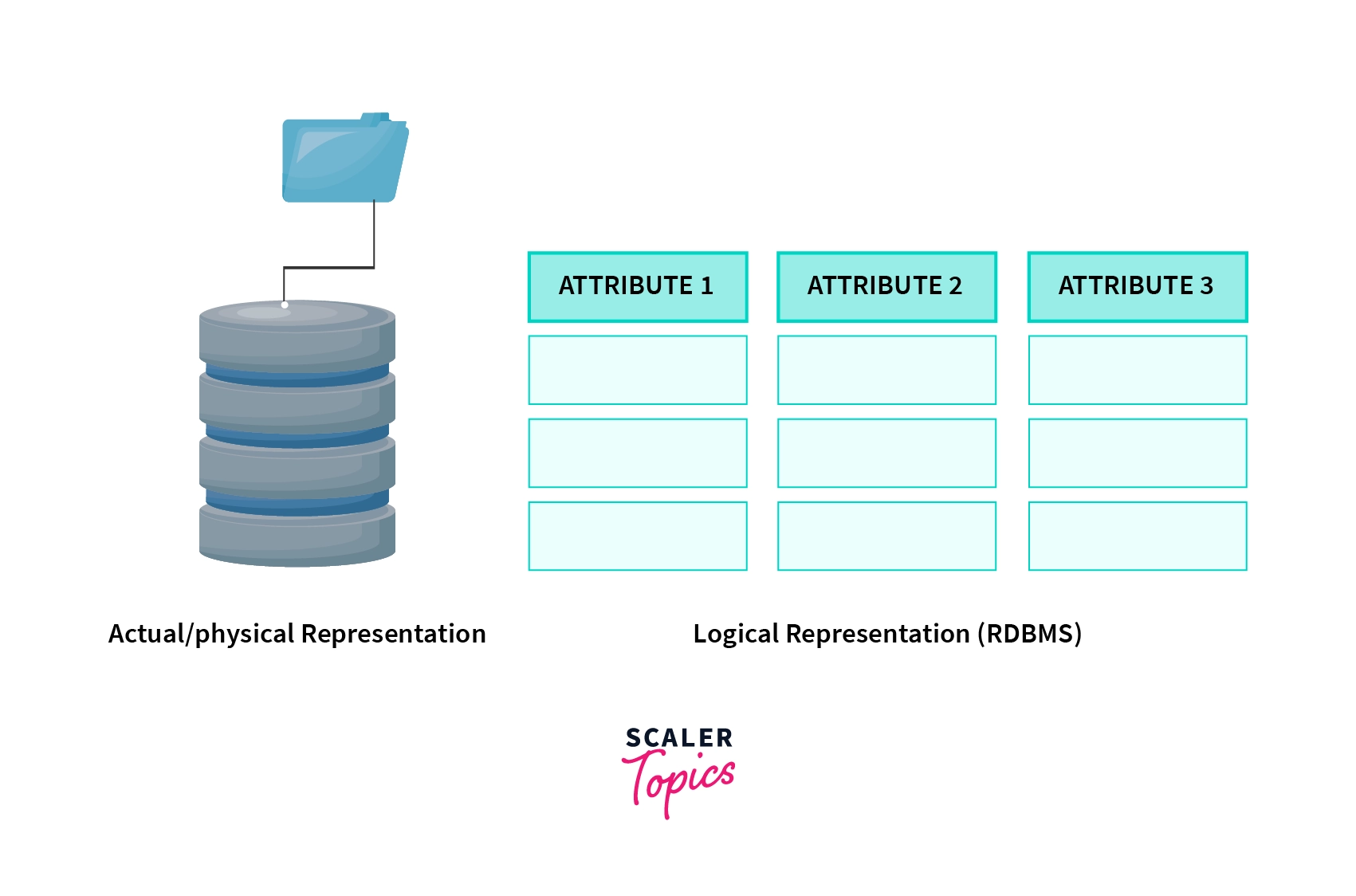In the case of RDBMS(Relational Database Management System), Data is logically represented in form of tables/relations. Similarly, in the case of the E-R Model, Data is logically represented in form of entities and relationships.

For Example, if we would like to store a student's detail, hence we will need a STUDENT table consisting of attributes such as roll number, name, course, etc.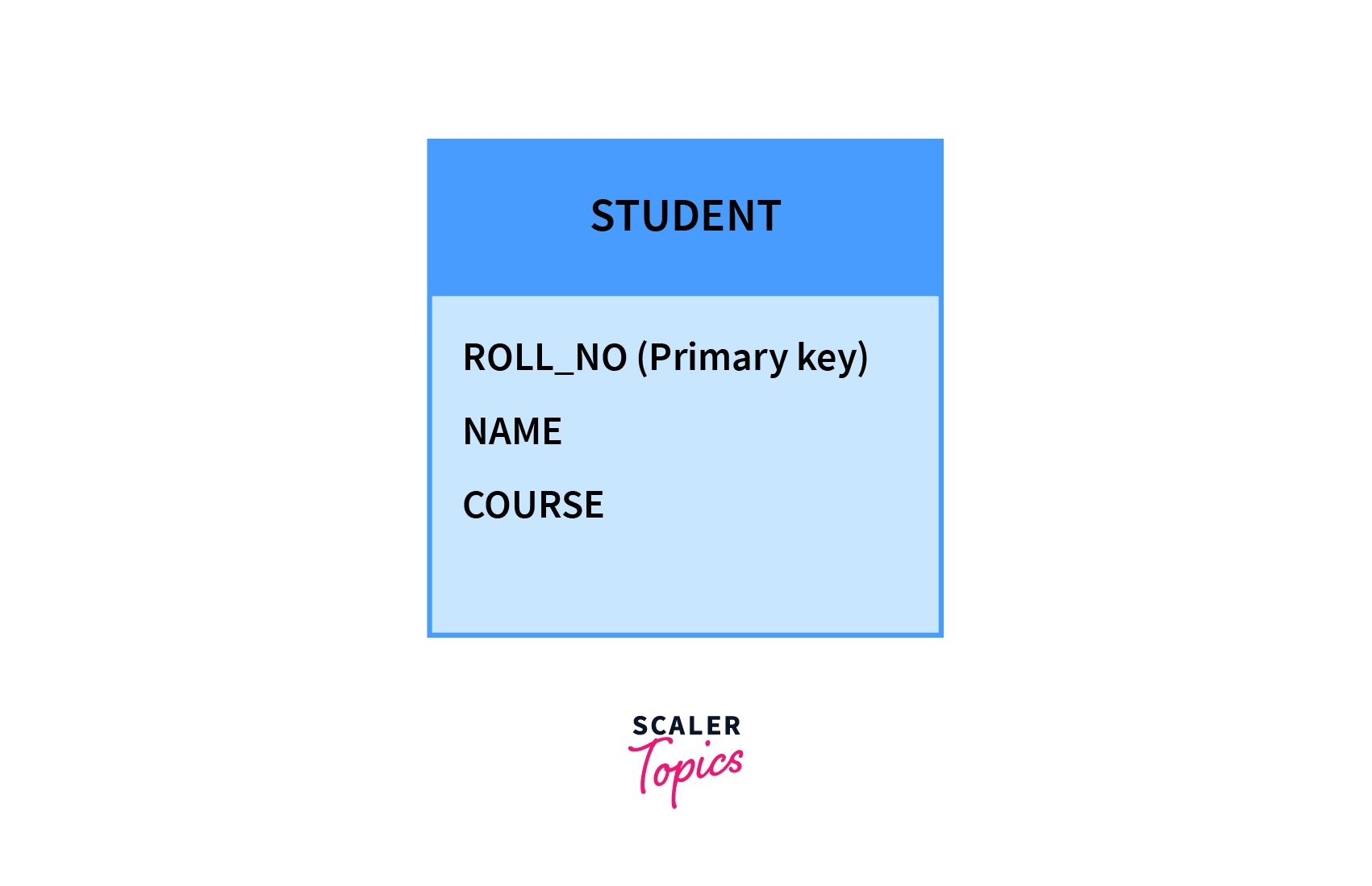The above Schema will be rendered as: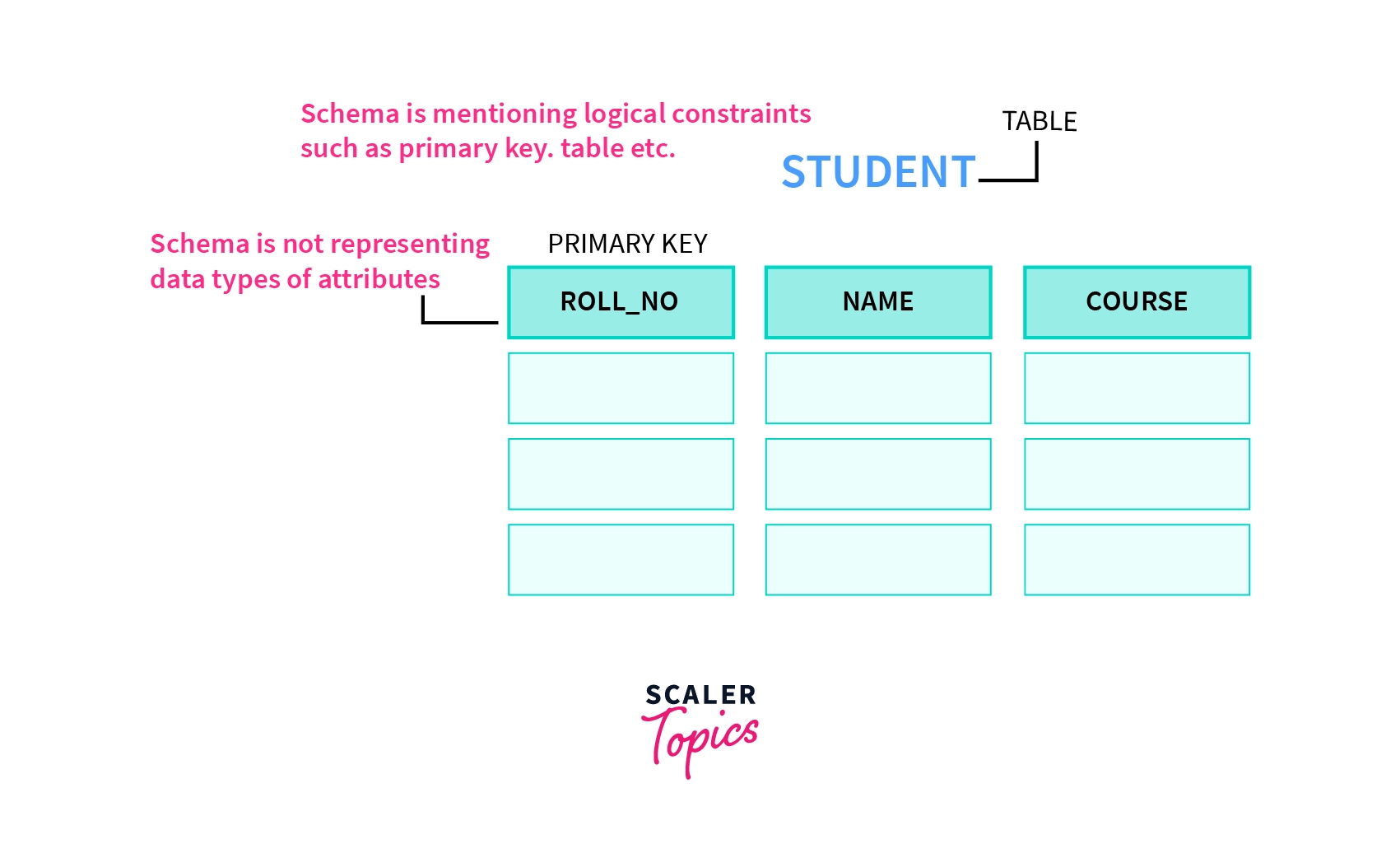## What is Schema in DBMS?

As you can see, these attributes create a structure/skeleton of the database and this structure is known as "schema".

Here, schema doesn't represent the constraints of the database such as data type used in the attributes. But it contains logical constraints such as Table, Primary Key, etc.

To break the direct contact between the user and the database.

Three Schema Architecture was introduced in 1970 and it consists of three levels: View, Logical, and Physical Level.

Three Schema Architecture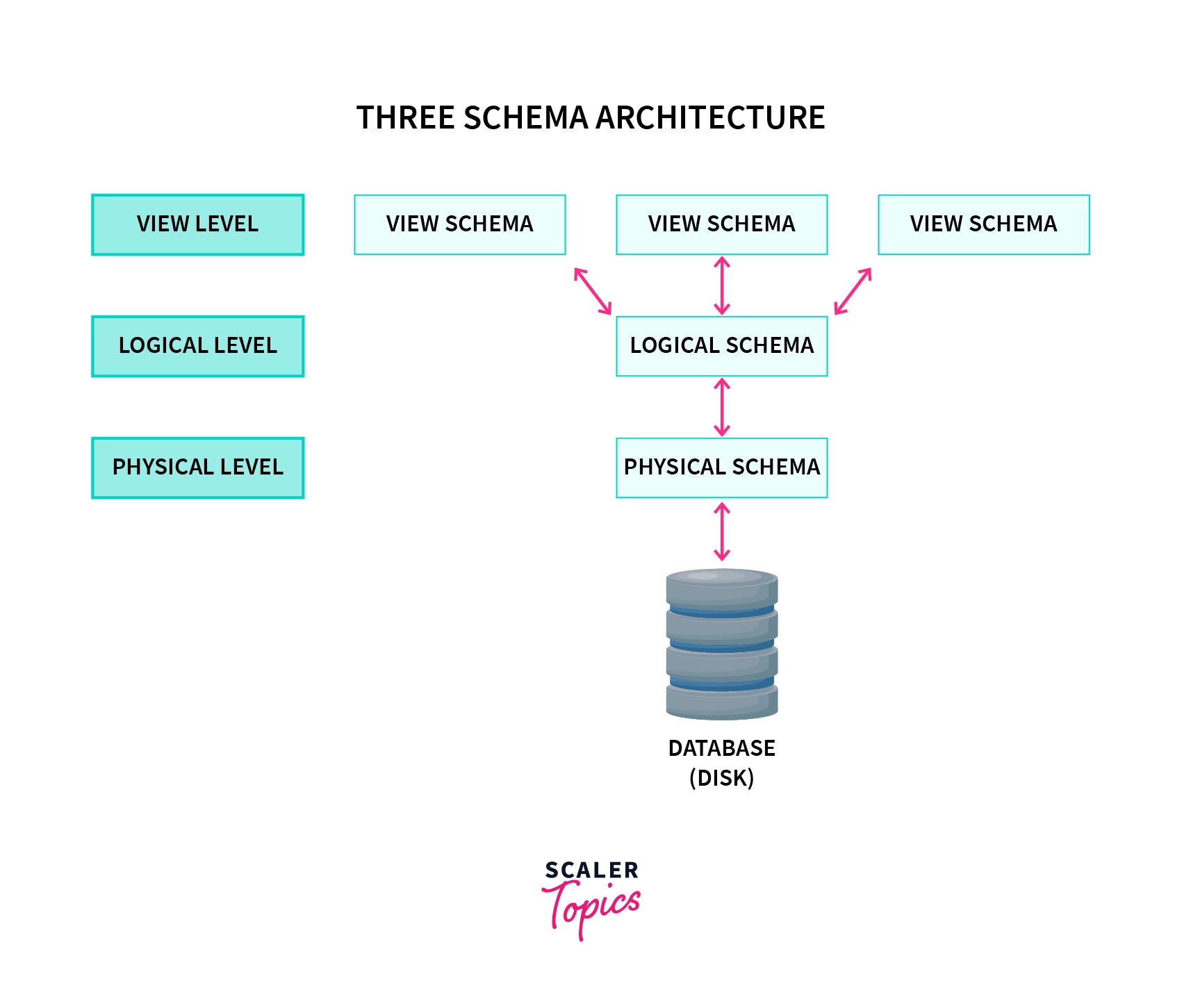### Physical Schema in DBMS

Physical Schema describes where the data is actually/physically present.

Here, the Database administrator decides where should be the data stored and how it should be stored in different blocks of storage.

### Logical Schema in DBMS

Logical Schema or also known as Conceptual Schema represents the structure of the database.

So for a student database, it describes the attributes/columns of the table. As discussed above, it also describes the logical constraints.

### View Schema in DBMS

View Schema or also known as External Schema is how the data is presented to a user.

Let's understand this with an example:

For a university's student management system, when a student logins into the system, the system shows Student details such as name, roll number, marks, department, year, etc.
These end-interactions with the database are described by View Schema in DBMS.

Screen showing details of a student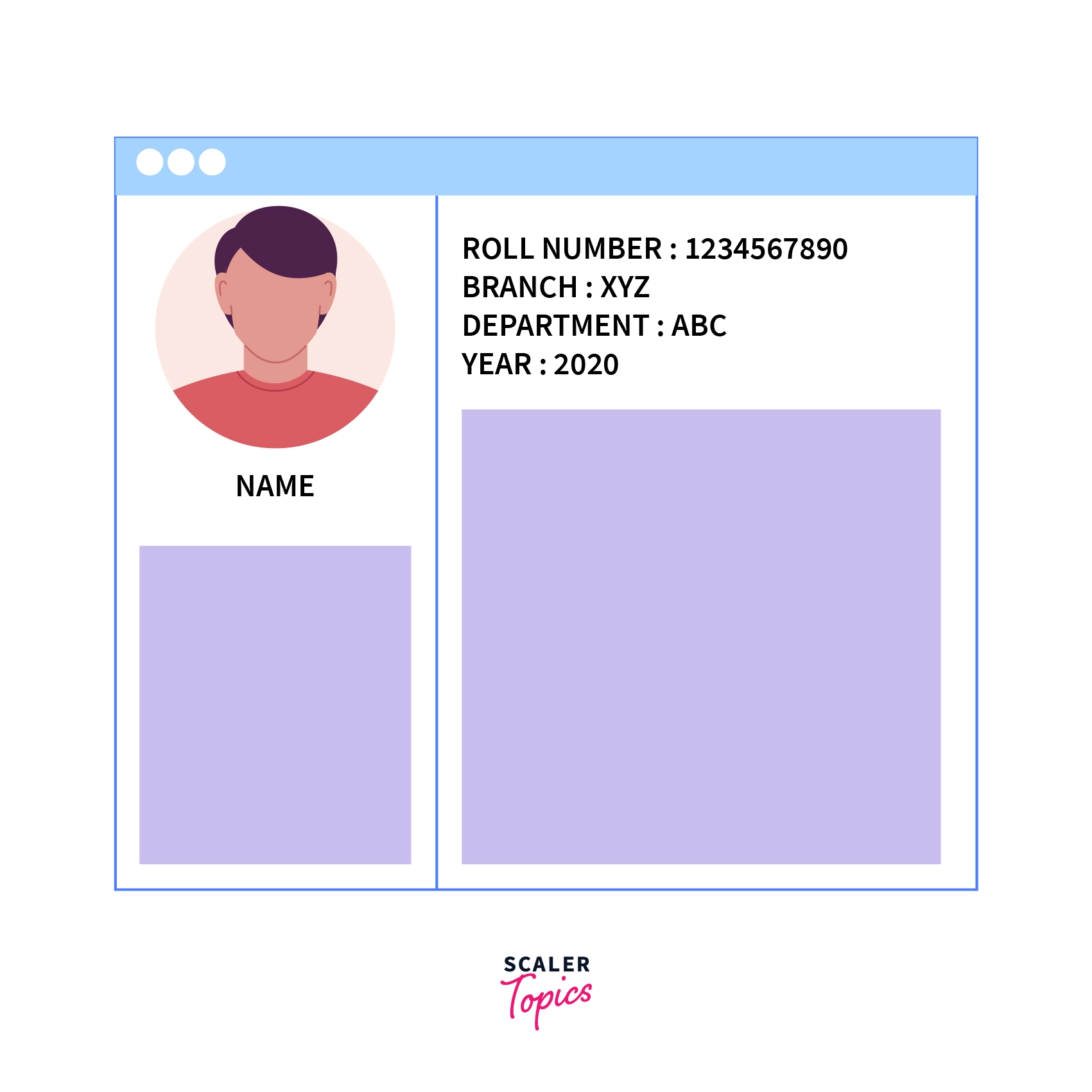But, Will this view be the same for every student?

Obviously not, that's why there are multiple View Schemas in View Level.

Different View Schmeas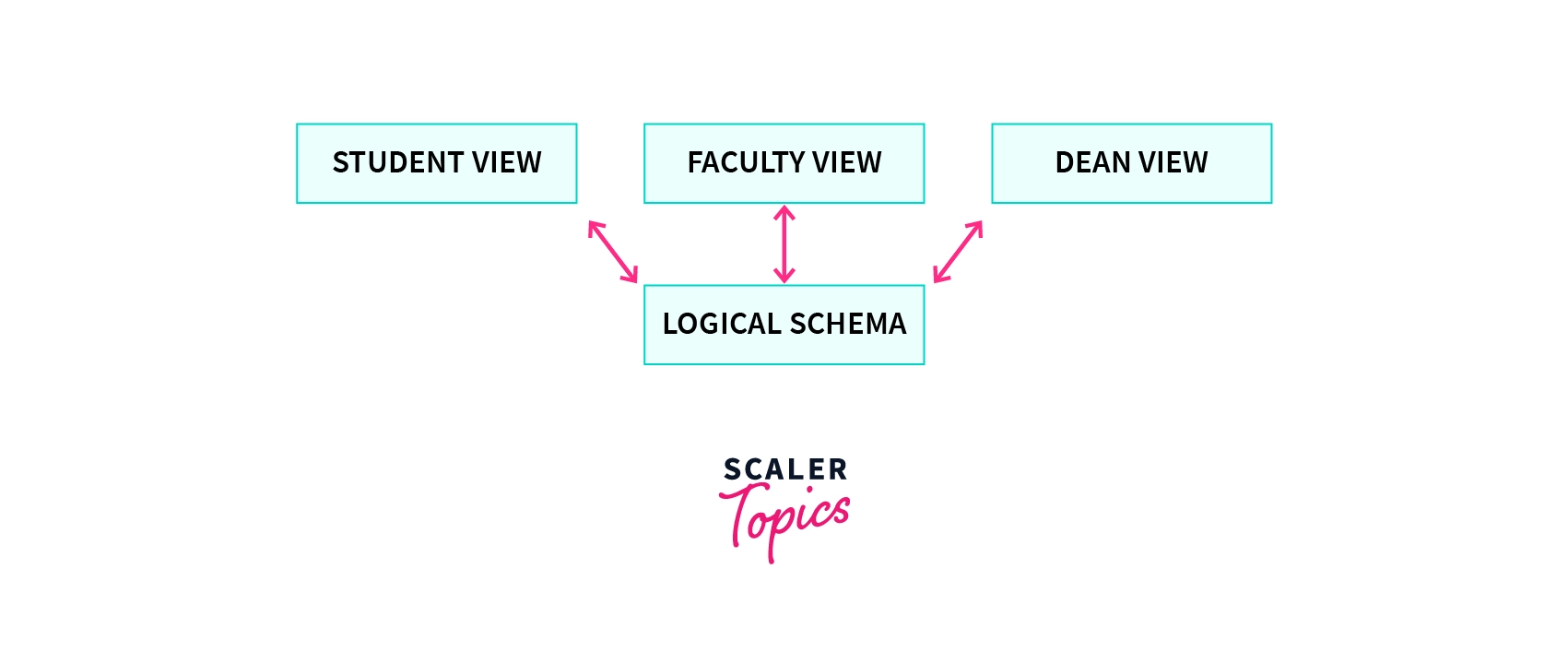## DBMS Instance

Schema is designed before creating the database and once the database is functional it is very difficult to update the schema as it represents the core structure of the database. Also Schema doesn't represent any data/information stored in the database.

In a database, there will be many read, and write operations. So we can't say we have content in the database as the data in the database is changing frequently.

So Instance in DBMS represents the data/information present in the database at a particular instance/time.

Let's say we have a STUDENT table, below is the representation of the table on 16th May.
Suppose a new student is enrolled in the university on 17th May. Now the database will be updated and this is shown below.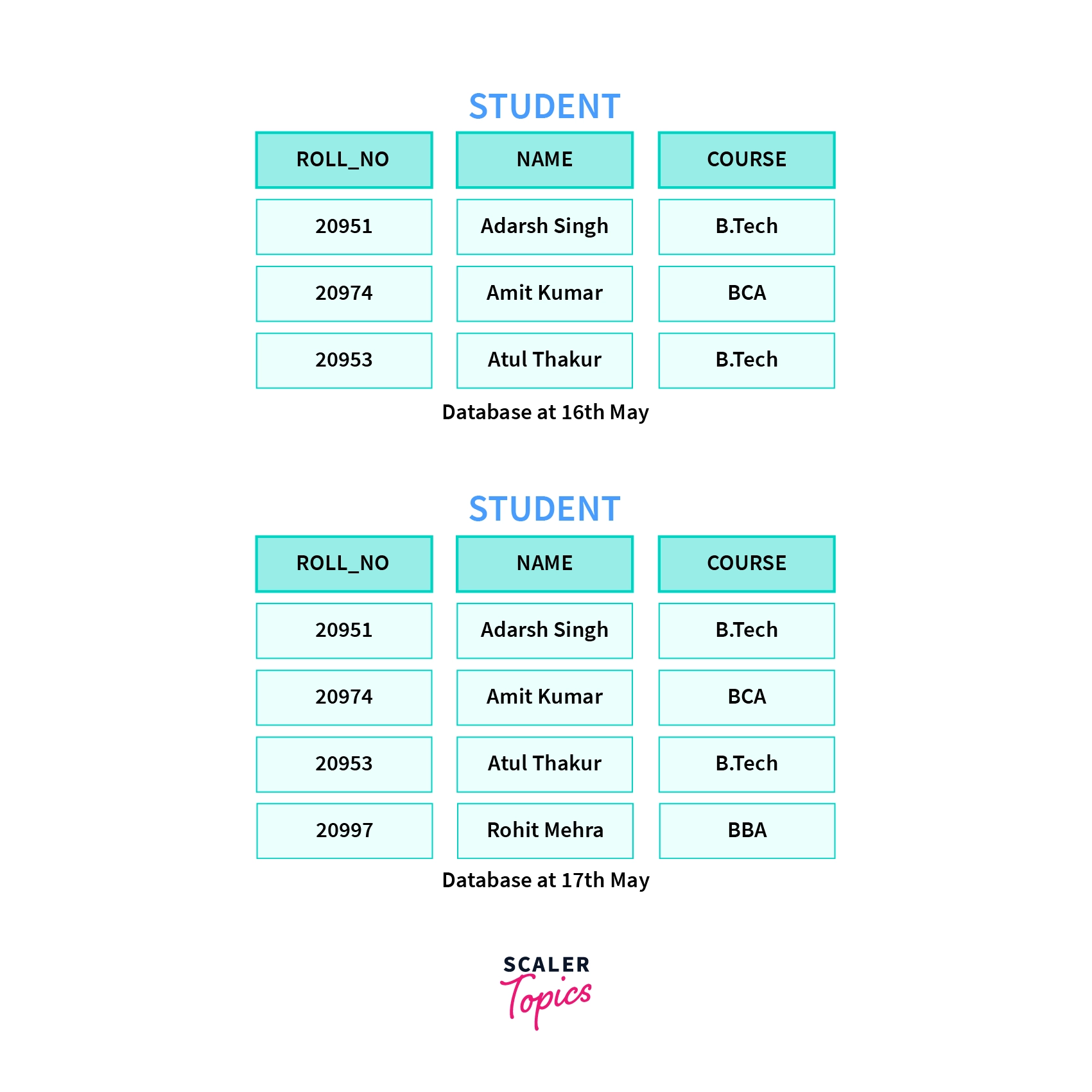Now, if we say we need instance of STUDENT table on 16th May, Table present at the left will be returned.

But, when we say we need instance of STUDENT table on 17th May, Table present on the right will be returned.

## Conclusion

• The Schema is the Structure of the database and it represents logical constraints such as Table, key, etc.
• To break direct contact between the user and the database, Three Schema Architecture was introduced and it consists of three levels: View, Logical and Physical Level.
• Since data stored in the database is changing frequently, Instance represents the data present in the database at a particular time.